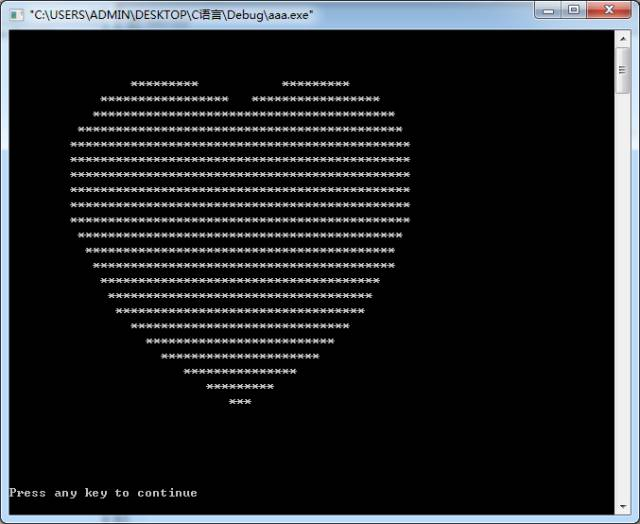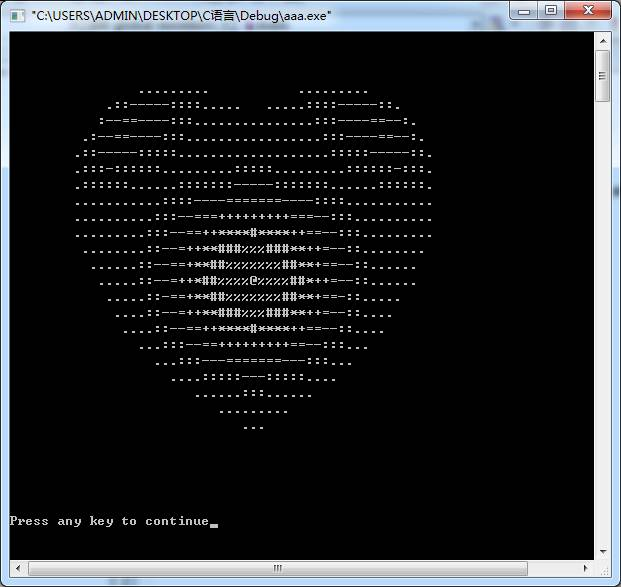2022
08-17

# 如何用c语言编写爱心```#include <stdio.h>

int main()

{

for (float y = 1.5f; y > -1.5f; y -= 0.1f)

{

for (float x = -1.5f; x < 1.5f; x += 0.05f)

{

float a = x * x + y * y - 1;

putchar(a * a * a - x * x * y * y * y <= 0.0f ? '*' : ' ');

}

putchar('\n');

}

}  ``````#include <stdio.h>

int main()

{

for (float y = 1.5f; y > -1.5f; y -= 0.1f)

{

for (float x = -1.5f; x < 1.5f; x += 0.05f) {

float z = x * x + y * y - 1;

float f = z * z * z - x * x * y * y * y;

putchar(f <= 0.0f ? ".:-=+*#%@"[(int)(f * -8.0f)] : ' ');

}

putchar('\n');

}

}  ```